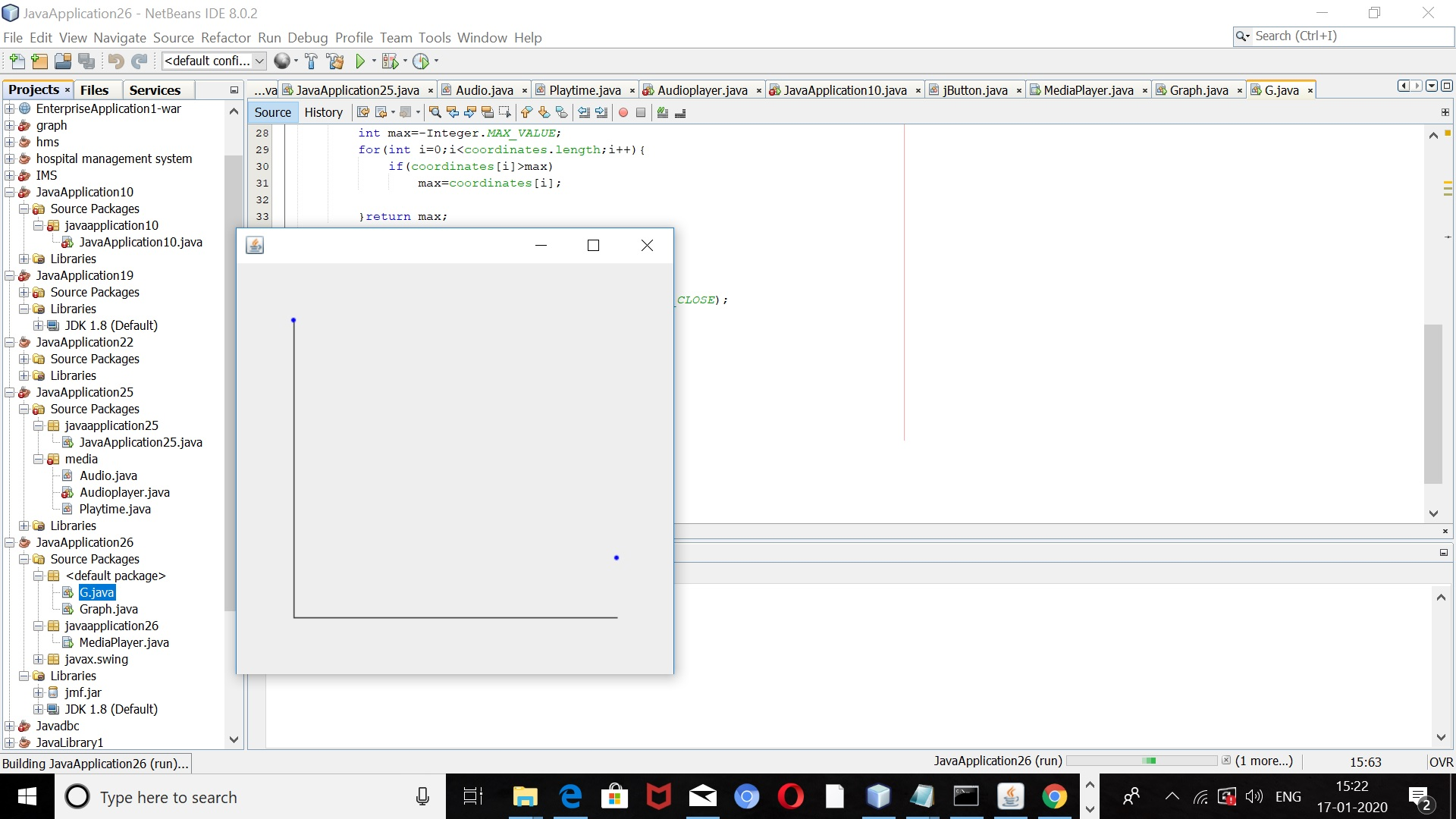# How to plot Graph in java

In this tutorial, we will learn how to draw a graph using Java. The drawing graph means plotting coordinates on a Cartesian plane. Co-ordinate is a combination of ordinate and abscissa ie(abscissa, ordinate). We can plot Graph using core Java using several topics ie. panels, graphics, AWT (Abstract Window Toolkit), etc.

## To plot a graph in Java

First of all, we will import all the required packages. The Required packages are

1. swing
2. awt
3. awt.geom

we import swing package for the use of JButtons, JPanel, JLabel, etc.

We import awt package, awt acronyms for Abstract Window Toolkit, awt enables the user to make a graphical user interface for front-end of the project.

We import awt.geom package for performing operations related to two-dimensional geometry. Two-dimensional geometry refers to geometry in which we use only the x-axis and y-axis. In three Dimensional geometry, we use three, x-axis, y-axis, z-axis.

Also, read: Simple Music Player In Java

## Code

See the code below:

```import java.awt.*;
import javax.swing.*;
import java.awt.geom.*;
public class G extends JPanel{
int[] coordinates={100,20};
int mar=50;
protected void paintComponent(Graphics g){
super.paintComponent(g);
Graphics2D g1=(Graphics2D)g;
g1.setRenderingHint(RenderingHints.KEY_ANTIALIASING,RenderingHints.VALUE_ANTIALIAS_ON);
int width=getWidth();
int height=getHeight();
g1.draw(new Line2D.Double(mar,mar,mar,height-mar));
g1.draw(new Line2D.Double(mar,height-mar,width-mar,height-mar));
double x=(double)(width-2*mar)/(coordinates.length-1);
double scale=(double)(height-2*mar)/getMax();
g1.setPaint(Color.BLUE);
for(int i=0;i<coordinates.length;i++){
double x1=mar+i*x;
double y1=height-mar-scale*coordinates[i];
g1.fill(new Ellipse2D.Double(x1-2,y1-2,4,4));
}

}
private int getMax(){
int max=-Integer.MAX_VALUE;
for(int i=0;i<coordinates.length;i++){
if(coordinates[i]>max)
max=coordinates[i];

}return max;
}

public static void main(String args[]){
JFrame frame =new JFrame();
frame.setDefaultCloseOperation(JFrame.EXIT_ON_CLOSE);
frame.setSize(400,400);
frame.setLocation(200,200);
frame.setVisible(true);
}
}```

G is the name of the class created, coordinates is the array of points to be plotted on the graph. We are extending JPanel to use it for representing the graph. JPanel is the light-weight container that is invisible. The default Layout of the panel is Flow-Layout. There are several layouts some of them are

1. grid layout
2. grid bag layout
3. flow layout
4. box layout
5. border layout
6. card layout
7. group layout
8. spring layout

But flow layout is the default layout for the panel.

### Functions and methods used in the project

We are using super.paintComponent(g), as we use the super keyword for calling parent class constructor or parent class method, And in this case, JPanel is the parent class.

Graphic 2D class again extends Graphics class to provide control over geometry. This is a fundamental class for two-dimensional shapes.

RenderingHints are the suggestions to the Java 2D about how it should perform its rendering.

In general, rendering means the way something is performed.

setRenderingHints() refer to the new set of hints that replaces the old ones.

We use Antialiasing for smoothing of the jagged edges in case of very low resolutions.

g1.draw() method is used to draw lines representing the x-axis and y-axis. Four coordinates are used to draw line (x1,y1,x2,y2).

Method setpaint() method is used to set the color to the points which we are plotting on the graph.

We calculate the value of co-ordinates using the method.

In for loop, we are using coordinates.length(), it is the method used to find the length of the array ie. coordinates.

The methods getWidth() and getHeight() are the methods of component that we use to return the height and width of the component we are using.

And x1 and y1 are the variables, that we use to plot points with respect to the size of the component instead of the cartesian plane.

### The setting of size and layout of the Frame

The frame is the object of JFrame and further, we perform four operations ie.

1. setsize
2. setLocation
3. setVisible
4. setDefaultCloseOperation

We use

• setLocation to set the location of the frame.
• setDefaultClose Operation to close the frame.
• setVisible to set the visibility of the frame.
• setSize to set the size of the frame.

The output result will show a graph like you can see below:### One response to “How to plot Graph in java”

1. eyequavy says:

hello, how can i solve this problem with this page showing? eyeg